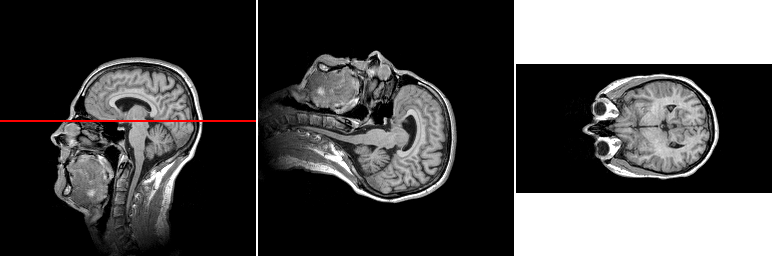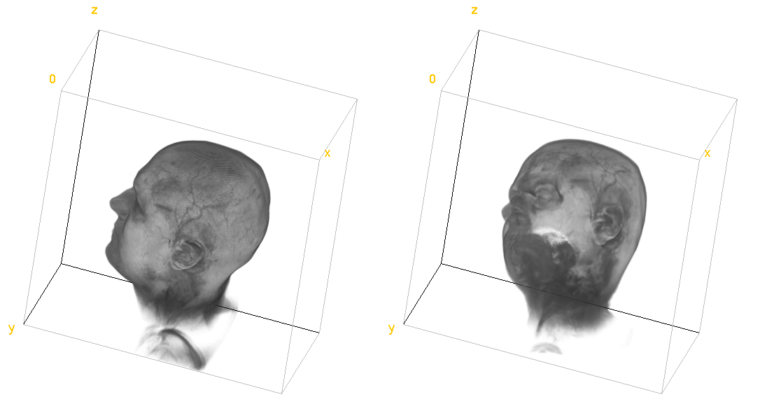# Image rotations¶

This example shows how to use rotate, rot90cw, rot90ccw, and reslice commands. First the example rotates input image 90 degrees clockwise, then 90 degrees around $$x$$-axis using the reslice command, and finally -60 degrees around vector $$[1, 1, 0]$$ using the rotate command.

def rotations():
"""
Demonstrates rotations and re-slicing.
"""

# Rotate 90 degrees clockwise (around z-axis)
rot90 = pi.newimage()
pi.rot90cw(img, rot90)
pi.writetif(rot90, output_file('rotate_90_clockwise'))

# Reslice (rotate 90 degrees around x- or y-axis)
top = pi.newimage()
pi.reslice(img, top, ResliceDirection.TOP)
pi.writetif(top, output_file('reslice_top'))

# General rotation.
# NOTE:
# - The size of the output must be set to desired value before the call
#   to rotate function.
# - The angle is given in radians. Here it is -60 degrees.
# - The axis is given as a vector and it does not need to be a unit vector.
grot = pi.newimage(img.get_data_type(), img.get_dimensions())
pi.rotate(img, grot, -60/180*3.14, [1, 1, 0])
pi.writetif(grot, output_file('general_rotation'))One slice of the input image (left), one slice of the input rotated 90 degrees clockwise (middle), and one slice of the input rotated 90 degrees around $$x$$-axis using the reslice command. The location of the slice in the rightmost panel is shown with red line in the leftmost panel.3D visualization of the original image (left) and the original rotated -60 degrees around $$[1, 1, 0]$$ (right).